2022-02-07
###### Out of Bag (OOB) score in Random Forests with example
2022-02-09

In this post, we will see how the Random Forest algorithm works internally. To truly appreciate it, it might be helpful to understand a bit about Decision-Tree Classifiers. But it’s not entirely required. We are not covering the pre-processing or feature creation steps involved in modeling — but only see what happens within the algorithm when we call the `.fit()` and `.transform()` methods for sklearn’s `RandomForestClassifier `package does.

Basically, Random Forest ( RF) is a tree-based algorithm. It is an ensemble of multiple random trees of different kinds. The final value of the model is the average of all the predictions/estimates created by each individual tree. We will be using the scikit-learn package, specifically the following modules:

`sklearn.ensemble.RandomForestClassifier`

( for the Random Forest Classifier algorithm found in the sklearn library )

`sklearn.ensemble.RandomForestRegressor`

(for the Random Forest regressor algorithm)

`sklearn.ensemble.RandomForestClassifier`

## Data

For illustration, we will be using training data similar to the one below.

`age ,glucose_level, weight, gender, smoking, ..., f98, f99` are all the independent variables or the features. `Diabetic `is the y-variable/dependent variable that we have to predict. The problem is to predict the patients who are likely to be diabetic

## Steps of Random Forest

With this basic information, let’s get started and understand what happens with we pass this training set to the algorithm.

### Step 1 — Bootstrapping

Once we provide the training data to the `RandomForestClassifier `model, it (the algorithm) selects a bunch of rows randomly. This process is called Bootstrapping (random replacement). For our example, let’s assume that it selects records.

The number of rows to be selected can be provided by the user in the hyper-parameter- `(max_samples)`:

```import sklearn.ensemble.RandomForestClassifier
my_rf = RandomForestClassifier(max_samples=100)
```

This only applies if we turn on bootstrapping in the hyper-parameter ( bootstrap = True). `bootstrap `is True by default. One row might get selected more than once

### Step 2 — Selecting features for sub-trees

Now, RF randomly selects a subset of features/columns. Here for the sake of simplicity and for the example, we are choosing 3 random features. We can control this number in the hyper-parameter — `max_features`:

```import sklearn.ensemble.RandomForestClassifier
my_rf = RandomForestClassifier(max_features=3)
```

### Step 3 — Selecting root node

Once the 3 random features are selected ( in our example), the algorithm runs a splitting of the m record (from step 1) and does a quick calculation of the before and after values of a metric. This metric could be either Gini-impurity or entropy. It is based on the criteria — Gini or entropy we set in the hyper-parameter.

```import sklearn.ensemble.RandomForestClassifier
my_rf = RandomForestClassifier(max_features=8 , criteria = 'gini')
criterion = 'gini' ( or 'entropy' )
```

By default, it is set to `criteria = 'gini’`. Whichever of the random feature split gives the least combined Gini impurity/ entropy value, that feature is selected as the root node. The records are split at this node based on the best splitting point.

### Step 4 — Selecting the child nodes

The algorithm performs the same process as in Step 2 and Step 4 and selects another set of 3 random features. ( 3 is the number we have specified — you can choose what you like — or leave it to the algorithm to choose the best number )

Based on the criteria ( `gini`/`entropy`), it selects which feature will go into the next node/child node, and further splitting of the records happens here.

### Step 5 —Further split and create child nodes

This process continues (Steps 2, 4) of selecting the random feature and splitting of the nodes happens till either of the following conditions happen:

• a) It runs out of the number of rows to split or has reached the threshold; a minimum number of rows to be present in each child node. This hyperparameter could be specified using `min_samples_leaf.`
• b) The Gini/entropy after splitting does not decrease beyond a minimum specified limit
• c) It has reached a specified number of splits ( `max_depth` )

We now have the first “mini-decision tree ”.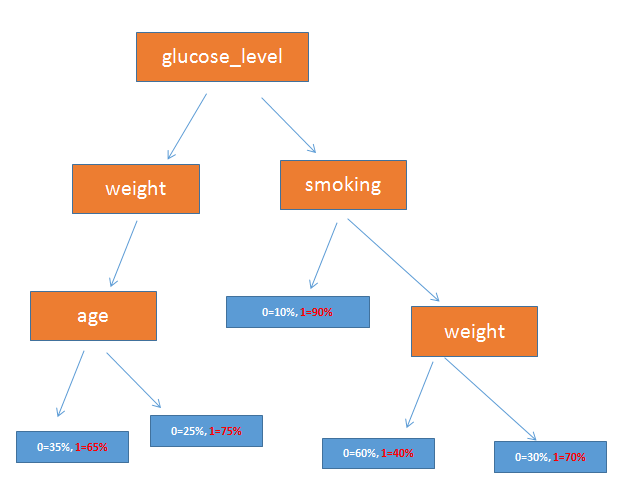The first mini-decision tree was created using the randomly selected rows ( records) and columns (features) (Image by Lars Nielsen)

### Step 6 — Create more mini-decision trees

The algorithm goes back to the data and does steps 1–5 to create the 2nd “mini-tree”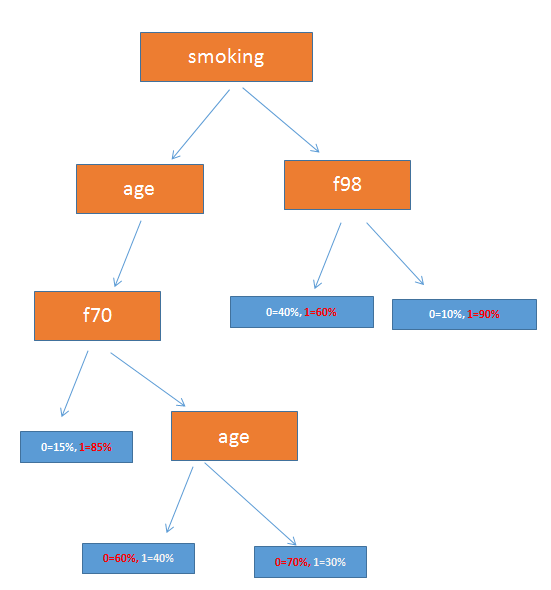This is the second mini tree that we created using another set of randomly chosen rows and columns. This mini-tree will have a different structure than the first one.

### Step 7. Build the forest of trees

Once the default value of 100 trees is reached (we now have 100 mini decision trees), the model is said to have completed its `fit()` process.

We can specify the number of trees we want to generate using the `n_estimators` hyper-parameter.

```import sklearn.ensemble.RandomForestClassifier
rf = RandomForestClassifier(n_estimators=300)
```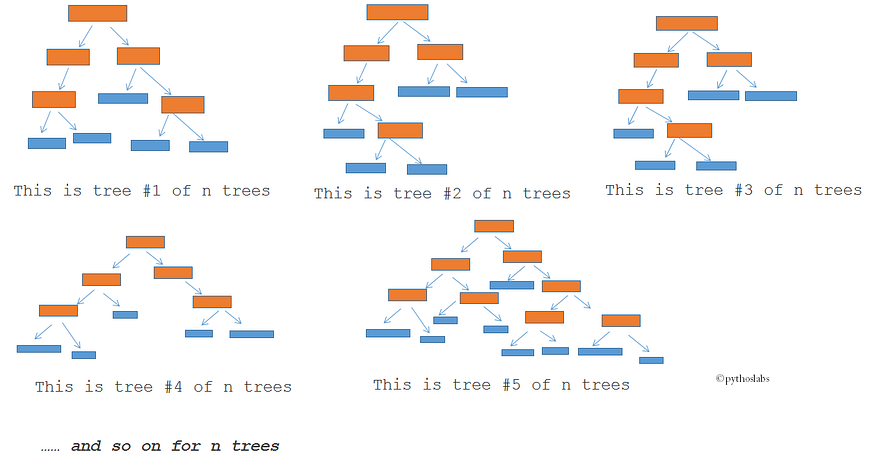we keep building the mini-trees till we have n trees ( n is the number specified by the n_estimators hyper-parameter, it has a default value of 100, if we do not specify anything). Here the blue boxes represent the end node.

Now we have a forest of randomly created mini-trees ( hence the name Random Forest )

### Step 7. Inferencing

Now let’s predict the values in an unseen data set ( the test data set ). For inferencing (more commonly referred to as predicting/scoring ) the test data, the algorithm passes the record through each mini-tree.

The values from the record traverse through the mini tree based on the variables that each node represents, and reach a leaf node ultimately. Based on the predetermined value of the leaf node (during training) where this record ends up, that mini-tree is assigned one prediction output. In the same manner, the same record goes through all the 100 mini-decision trees and each of the 100 trees has a prediction output for that record.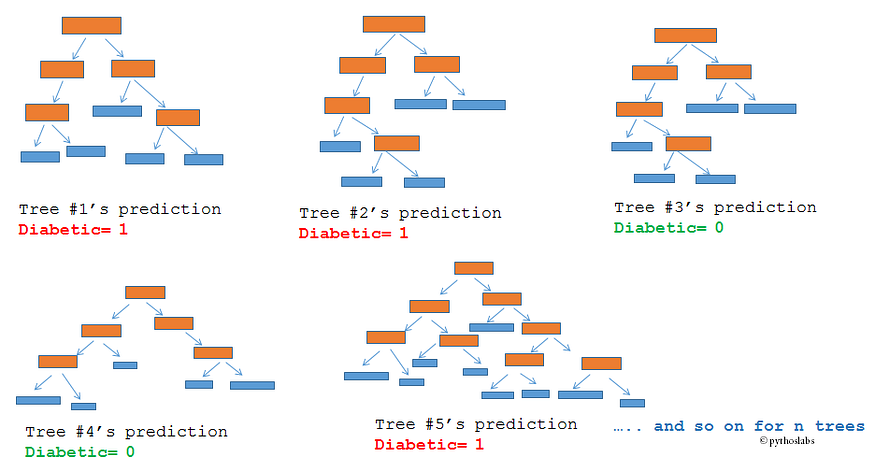All the mini-trees make one prediction for that record. (Image by Lars Nielsen)

The final prediction value for this record is calculated by taking simple voting of these 100 mini trees.

Now we have the prediction for a single record.

The algorithm iterates through all the records of the test set following the same process and does a calculation of the overall accuracy!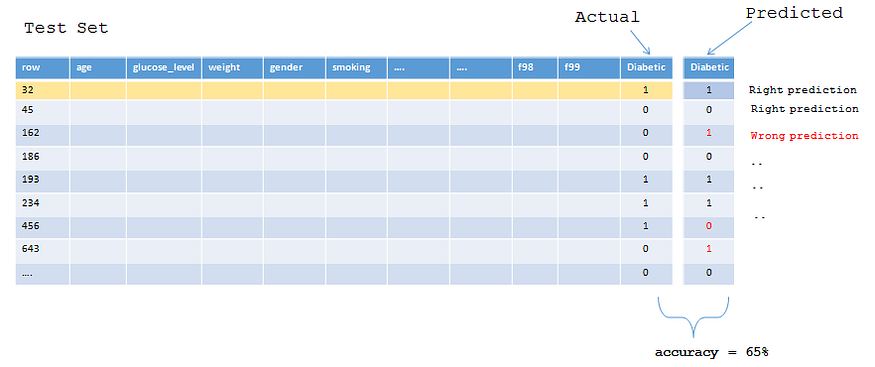Iterate the process of obtaining the prediction for each row of the test set to arrive at the final accuracy.

## Random Forest Classifier — hyperparameters

Now let’s get a deeper understanding of what each of the parameters does in the Random Forest algorithm.

1. `n_estimators` ( default = `100` )

Since the RandomForest algorithm is an ensemble modeling technique, it ‘increases the generalization’ by creating a number of different kinds of trees with different depths and sizes. n_estimators is the number of trees we want the algorithm to create. Increasing the number of trees in the forest decreases the variance of the overall model and doesn’t contribute to overfitting. From the standpoint of generalization performance, using more trees is therefore better.

2. `criterion` (default = `gini`)

The measure to determine where/on what features a tree has to be split can be determined by two methods — by calculating the Gini-impurity or by entropy. For example, suppose there are two parameters `gender` and `nationality` based on which the splitting of the tree has to be done. The algorithm does the split using both the features and chooses the one which results in a lower entropy or a lower Gini-impurity after the split as the feature to be split on, discarding the other

3. `max_depth` (default = `None`)

is the measure of how much further the tree has to be expanded down to each node till we get to the leaf node. Generally in a tree-based algorithm, the more the depth, the more the chance that it overfits the data. Since Random Forest ensembles several different trees together, it is generally accepted to have deep trees.

4. `min_samples_split` (default = `2`)

We can specify the minimum number of elements/records that has to be present in each node to determine if the algorithm can stop splitting further. If we mention the `min_sample_split` to be 60, after 4 splits if the node still has more than 60 elements or records, it is a potential candidate to be split further. i.e. the splitting continues as long as there are more than 60 records.

5. `max_features` (default = `auto`)

At every split, the algorithm chooses some features ( randomly ) to be based on which the tree starts to split. `max_features `determines how many features need to be selected for determining the split. Considering more features increases the chance of finding a better split. But, it also increases the correlation between trees, increasing the variance of the overall model. Recommended default values are the square root of the total number of features for classification problems, and 1/3 of the total number for regression problems. As with tree size, it may be possible to increase performance by tuning.

There are multiple options available in Scikit-Learn to assign maximum features. Here are a few of them :

1. Auto/None: This will simply take all the features which make sense in every tree. Here we simply do not put any restrictions on the individual tree.
2. sqrt: This option will take the square root of the total number of features in an individual run. For instance, if the total number of variables is 100, we can only take 10 of them in an individual tree. ”log2″ is another similar type of option for max_features.
3. 0.2: This option allows the random forest to take 20% of variables in an individual run. We can assign and value in a format “0.x” where we want x% of features to be considered.

How does “max_features” impact performance and speed?

Increasing max_features generally improves the performance of the model as at each node now we have a higher number of options to be considered. However, this is not necessarily true as this decreases the diversity of individual trees which is the USP of random forest. But, for sure, you decrease the speed of the algorithm by increasing the max_features. Hence, you need to strike the right balance and choose the optimal max_features.

6. `bootstrap` (default = `True`)

Once we provide the training data to the `RandomForestClassifier `model thalgorithm selects a bunch of rows randomly with replacement to build the trees. This process is called Bootstrapping (Random replacement). If the bootstrap option is set to `False`, no random selection happens and the whole dataset is used to create the trees.

### Sklearn parameter that will make the model training easier

There are a few attributes that have a direct impact on model training speed. Following are the key parameters that we can tune for model speed:

#### n_jobs

This parameter tells the engine how many processors is it allowed to use. A value of “-1” means there is no restriction whereas a value of “1” means it can only use one processor. Here is a simple experiment we can do with Python to check this metric:

```%timeit
model = RandomForestRegressor(n_estimator = 100, oob_score = TRUE,n_jobs = 1,random_state =1)
model.fit(X,y)
# Output:  1 loop best of 3 : 1.7 sec per loop

%timeit
model = RandomForestRegressor(n_estimator = 100,oob_score = TRUE,n_jobs = -1,random_state =1)
model.fit(X,y)
# Output: 1 loop best of 3 : 1.1 sec per loop
```

#### random_state

This parameter makes a solution easy to replicate. A definite value of random_state will always produce same results if given with the same parameters and training data. I have personally found an ensemble with multiple models of different random states and all optimum parameters sometimes perform better than the individual random states.

#### oob_score

This is a random forest cross-validation method. It is very similar to the leave one out validation technique, however, this is so much faster. This method simply tags every observation used in different tress. And then it finds out a maximum vote score for every observation based on only trees which did not use this particular observation to train themselves.

Here is a single example of using all these parameters in a single function :

```model = RandomForestRegressor(n_estimator = 100, oob_score = TRUE, n_jobs = -1,random_state =50,                                         max_features = "auto", min_samples_leaf = 50)
model.fit(X,y)
```

## Overfitting in Random Forests

In this section, we discuss everything you need to know about random forest models and overfitting. We start with a discussion of what overfitting is and how to determine when a model is overfitted. After that, we discuss random forests and the likelihood that random forest models will overfit.

## Overfitting

Overfitting is a common phenomenon we should look out for any time we are training a machine learning model. Overfitting happens when a model pays too much attention to the specific details of the dataset that it was trained on. Specifically, the model picks up on patterns that are specific to the observations in the training data but do not generalize to other observations. The model is able to make great predictions on the data it was trained on but is not able to make good predictions on data it did not see during training.

Overfitting is a problem because machine learning models are generally trained with the intention of making predictions on unseen data. Models that have overfit to their training data set are not able to make good predictions on new data that they did not see during training, so they are not able to make predictions on unseen data.

## How to recognize overfitting?

If you plan to use a machine learning model to make predictions on unseen data, you should always check to make sure that your model is not overfitting to the training data. How do we check whether the model is overfitting to the training data?

In order to check whether a model is overfitting to the training data, we should make sure to split the dataset into a training dataset that is used to train the model and a test dataset that is not touched at all during model training. This way we will have a dataset available that the model did not see at all during training that we can use to assess whether our model is overfitting.

We should generally allocate around 70% of data to the training dataset and 30% of data to the test dataset. Only after we trained the model on the training dataset and optimized hyper parameters, we plan to optimize on the test dataset. At that point, we can use the model to make predictions on both the test data and the training data and then compare the performance metrics on the test and training data.

If a model is overfitting to the training data, we will notice that the performance metrics on the training data are much better than the performance metrics on the test data.

## Overfitting in random forests

In general, random forests are much less likely to overfit than other models because they are made up of many weak classifiers that are trained completely independently on completely different subsets of the training data. Random forests are a great option to spring for if we want to train a quick model that is not likely to overfit. That being said, it is possible that a random forest model might overfit in some cases so we should still make sure to look out for overfitting when we train random forest models.

## Prevent overfitting in random forests

Here are some easy ways to prevent overfitting in random forests.

• Reducing tree depth. If you do believe that your random forest model is overfitting, the first thing you should do is reduce the depth of the trees in the random forest model. Different implementations of random forest models will have different parameters that control this, but generally there will be a parameter that explicitly controls the number of levels deep a tree can get, the number of splits a tree can have, or the minimum size of the terminal nodes. Reducing model complexity generally ameliorates overfitting problems and reducing tree depth is the easiest way to reduce complexity in random forests.
• Reducing the number of variables sampled at each split. We can also reduce the number of variables considered for each split to introduce more randomness into the model. To take a step back, each time a split is created in a tree, a subset of variables is taken and only those variables are considered to be the variable that is split on. If we consider all or most of the variables at each split, trees may all end up looking the same because the same splits on the same variables are chosen. If we consider a smaller subset of variables at each split, the trees are less likely to look the same because it is unlikely that the same variables were even available for consideration at each split.
• Using more data. Finally, we can always try increasing the size of the dataset. Overfitting is more likely to happen when complex models are trained on small datasets so increasing the size of the dataset may help.

RandomForests can be regularized by tweaking the following parameters:

• Decreasing `max_depth`: This is a parameter that controls the maximum depth of the trees. The bigger it is, there more parameters will have, remember that overfitting happens when there’s an excess of parameters being fitted.
• Increasing `min_samples_leaf`: Instead of decreasing `max_depth` we can increase the minimum number of samples required to be at a leaf node, this will limit the growth of the trees too and prevent having leaves with very few samples (Overfitting!)
• Decreasing `max_features`: As previously mentioned, overfitting happens when there’s abundance of parameters being fitted, the number of parameters hold a direct relationship with the number of features in the model, therefore limiting the amount of features in each tree will prove valuable to help control overfitting.

Resources:

https://towardsdatascience.com/a-pictorial-guide-to-understanding-random-forest-algorithm-fbf570a0ae0d

https://medium.com/swlh/understanding-the-random-forest-function-parameters-in-scikit-learn-9f42fde0101

https://towardsdatascience.com/random-forest-hyperparameters-and-how-to-fine-tune-them-17aee785ee0d

https://medium.com/all-things-ai/in-depth-parameter-tuning-for-random-forest-d67bb7e920d

https://www.section.io/engineering-education/hyperparmeter-tuning/

https://towardsdatascience.com/optimizing-hyperparameters-in-random-forest-classification-ec7741f9d3f6##### Amir Masoud Sefidian
Machine Learning Engineer